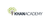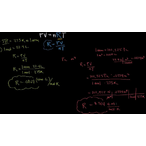# Class 11 Physics (India): Derivation of gas constants using molar volume and STP

Product type
Total time

## Class 11 Physics (India): Derivation of gas constants using molar volume and STPKhan Academy

Need more information? Get more details on the site of the provider.## Class 11 Physics (India)

Let's learn, practice, and master topics of class 11 physics (NCERT) starting with kinematics and then moving to dynamics with Newton's laws of motion, work, energy, and power. Let's then use these as the foundation to learn about centre of mass, rotational motion, gravitation, solids, fluids, thermodynamics, and oscillations and waves.

## Ideal gas equation

In this tutorial, Sal shows you how to use the ideal gas equation in calculations.

## Topic: Science

Learn about all the sciences, from physics, chemistry and biology, to cosmology and astronomy, across hundreds of videos, articles and practice questions.

There are no frequently asked questions yet. If you have any more questions or need help, contact our customer service.

Didn't find what you were looking for? See also: Oil & Gas, General Management, Software / System Engineering, Health Management, and Gas Engineering.

## Class 11 Physics (India)

Let's learn, practice, and master topics of class 11 physics (NCERT) starting with kinematics and then moving to dynamics with Newton's laws of motion, work, energy, and power. Let's then use these as the foundation to learn about centre of mass, rotational motion, gravitation, solids, fluids, thermodynamics, and oscillations and waves.

## Ideal gas equation

In this tutorial, Sal shows you how to use the ideal gas equation in calculations.

## Topic: Science

Learn about all the sciences, from physics, chemistry and biology, to cosmology and astronomy, across hundreds of videos, articles and practice questions.

There are no reviews yet.
• View related products with reviews: Oil & Gas.